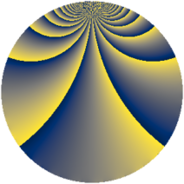# Properties

 Label 512.2.gLevel $512$ Weight $2$ Character orbit 512.g Rep. character $\chi_{512}(65,\cdot)$ Character field $\Q(\zeta_{8})$ Dimension $48$ Newform subspaces $8$ Sturm bound $128$ Trace bound $5$

# Related objects

## Defining parameters

 Level: $$N$$ $$=$$ $$512 = 2^{9}$$ Weight: $$k$$ $$=$$ $$2$$ Character orbit: $$[\chi]$$ $$=$$ 512.g (of order $$8$$ and degree $$4$$) Character conductor: $$\operatorname{cond}(\chi)$$ $$=$$ $$32$$ Character field: $$\Q(\zeta_{8})$$ Newform subspaces: $$8$$ Sturm bound: $$128$$ Trace bound: $$5$$ Distinguishing $$T_p$$: $$3$$, $$5$$

## Dimensions

The following table gives the dimensions of various subspaces of $$M_{2}(512, [\chi])$$.

Total New Old
Modular forms 320 80 240
Cusp forms 192 48 144
Eisenstein series 128 32 96

## Trace form

 $$48 q + 16 q^{9} + O(q^{10})$$ $$48 q + 16 q^{9} + 16 q^{25} - 32 q^{33} + 16 q^{41} + 16 q^{57} - 32 q^{65} + 16 q^{73} + 16 q^{89} - 32 q^{97} + O(q^{100})$$

## Decomposition of $$S_{2}^{\mathrm{new}}(512, [\chi])$$ into newform subspaces

Label Dim. $$A$$ Field CM Traces $q$-expansion
$$a_2$$ $$a_3$$ $$a_5$$ $$a_7$$
512.2.g.a $$4$$ $$4.088$$ $$\Q(\zeta_{8})$$ None $$0$$ $$-4$$ $$-8$$ $$-4$$ $$q+(-1-\zeta_{8}^{3})q^{3}+(-2-2\zeta_{8}+\zeta_{8}^{2}+\cdots)q^{5}+\cdots$$
512.2.g.b $$4$$ $$4.088$$ $$\Q(\zeta_{8})$$ None $$0$$ $$-4$$ $$8$$ $$4$$ $$q+(-1-\zeta_{8}^{3})q^{3}+(2+2\zeta_{8}-\zeta_{8}^{2}+\cdots)q^{5}+\cdots$$
512.2.g.c $$4$$ $$4.088$$ $$\Q(\zeta_{8})$$ None $$0$$ $$4$$ $$-8$$ $$4$$ $$q+(1+\zeta_{8}^{3})q^{3}+(-2-2\zeta_{8}+\zeta_{8}^{2}+\cdots)q^{5}+\cdots$$
512.2.g.d $$4$$ $$4.088$$ $$\Q(\zeta_{8})$$ None $$0$$ $$4$$ $$8$$ $$-4$$ $$q+(1+\zeta_{8}^{3})q^{3}+(2+2\zeta_{8}-\zeta_{8}^{2}+\zeta_{8}^{3})q^{5}+\cdots$$
512.2.g.e $$8$$ $$4.088$$ 8.0.18939904.2 None $$0$$ $$-4$$ $$-8$$ $$8$$ $$q+(-1+\beta _{4}-\beta _{5})q^{3}+(-1-\beta _{6})q^{5}+\cdots$$
512.2.g.f $$8$$ $$4.088$$ 8.0.18939904.2 None $$0$$ $$-4$$ $$8$$ $$-8$$ $$q+(-1+\beta _{4}-\beta _{5})q^{3}+(1+\beta _{6})q^{5}+\cdots$$
512.2.g.g $$8$$ $$4.088$$ 8.0.18939904.2 None $$0$$ $$4$$ $$-8$$ $$-8$$ $$q+(1+\beta _{3}-\beta _{4}+\beta _{7})q^{3}+(-1-\beta _{4}+\cdots)q^{5}+\cdots$$
512.2.g.h $$8$$ $$4.088$$ 8.0.18939904.2 None $$0$$ $$4$$ $$8$$ $$8$$ $$q+(1+\beta _{3}-\beta _{4}+\beta _{7})q^{3}+(1+\beta _{4})q^{5}+\cdots$$

## Decomposition of $$S_{2}^{\mathrm{old}}(512, [\chi])$$ into lower level spaces

$$S_{2}^{\mathrm{old}}(512, [\chi]) \cong$$ $$S_{2}^{\mathrm{new}}(32, [\chi])$$$$^{\oplus 5}$$$$\oplus$$$$S_{2}^{\mathrm{new}}(128, [\chi])$$$$^{\oplus 3}$$$$\oplus$$$$S_{2}^{\mathrm{new}}(256, [\chi])$$$$^{\oplus 2}$$